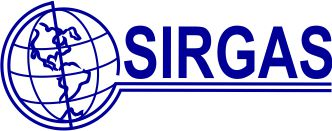Sistema de Referencia Geocéntrico para las Américas (SIRGAS)## Use of velocities in the processing of GNSS data

The GNSS data processing requires the reference station positions in the same reference frame and at the same epoch as the satellite orbits. The weekly station positions of the SIRGAS Continuously Operating Stations (SIRGAS-CON network) satisfy these requirements and therefore, it is highly recommended to use SIRGAS-CON stations and their weekly coordinates as reference points for precise GNSS positioning in the SIRGAS Region. If the reference points in a given GNSS surveying are not SIRGAS-CON stations, the following is needed:

1. Transformation of the station reference positions to the same reference frame, in which the satellite orbits are given. For instance, at present, the SIRGAS95 coordinates shall be transformed from the ITRF94 to the ITRF2014 (IGb14). In the same manner, the SIRGAS2000 coordinates have to be transformed from ITRF2000 to ITRF2014 (IGb14) (see transformation parameters between different ITRFs).

2. Reduction of the reference positions from the realization epoch to the observation epoch; i.e. the coordinates referred to SIRGAS95 shall be reduced from 1995.4 to that day, at the GNSS positioning was performed, for instance, 2018.0. Similarly, the SIRGAS2000 associated coordinates must be reduced from 2000.4 to 2018.0. This reduction is carried out through:

X (t) = X (t0) + (t - t0) * Vx  ;  Y (t) = Y (t0) + (t - t0) * Vy  ;  Z (t) = Z (t0) + (t - t0) * Vz

being X(t), Y(t), Z(t) the station positions at the desired epoch, X(t0), Y(t0), Z(t0) the reference coordinates, (t - t0) the time interval between the reference epoch and the observation epoch, and Vx, Vy, Vz the velocities of the reference stations.

3. It is desirable that the velocities of reference stations are derived from iterative GNSS measurements covering a minimum time span of two years. If this is not possible, and the velocities of the reference stations are unknown, Vx, Vy, Vz can be inferred from the VEMOS model.

4.  Once the reference station positions are related to the observation epoch, the positions of the new GNSS points can be determined.

5. The coordinates of the new points shall be reduced to the reference epoch (see item 2).

6. Finally, the new coordinates must be transformed into the official reference frame, i.e. SIRGAS95 (ITRF94), SIRGAS2000 (ITRF2000), etc. Velocities applied for reducing the new station positions to the reference epoch shall be stored together with those positions. GNSS points including VEMOS velocities should not be classified as reference stations.

More details in: Procesamiento de información GPS con relación a marcos de referencia de épocas diferentes (H. Drewes).

It should keep in mind that co-seismic effects (jumps) affecting station positions must be considered in additional analyses.